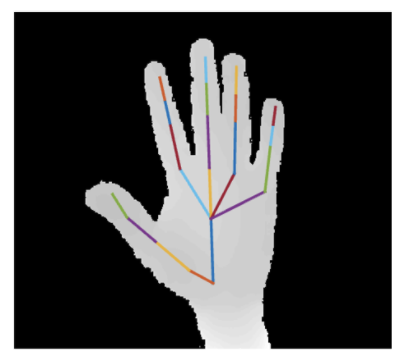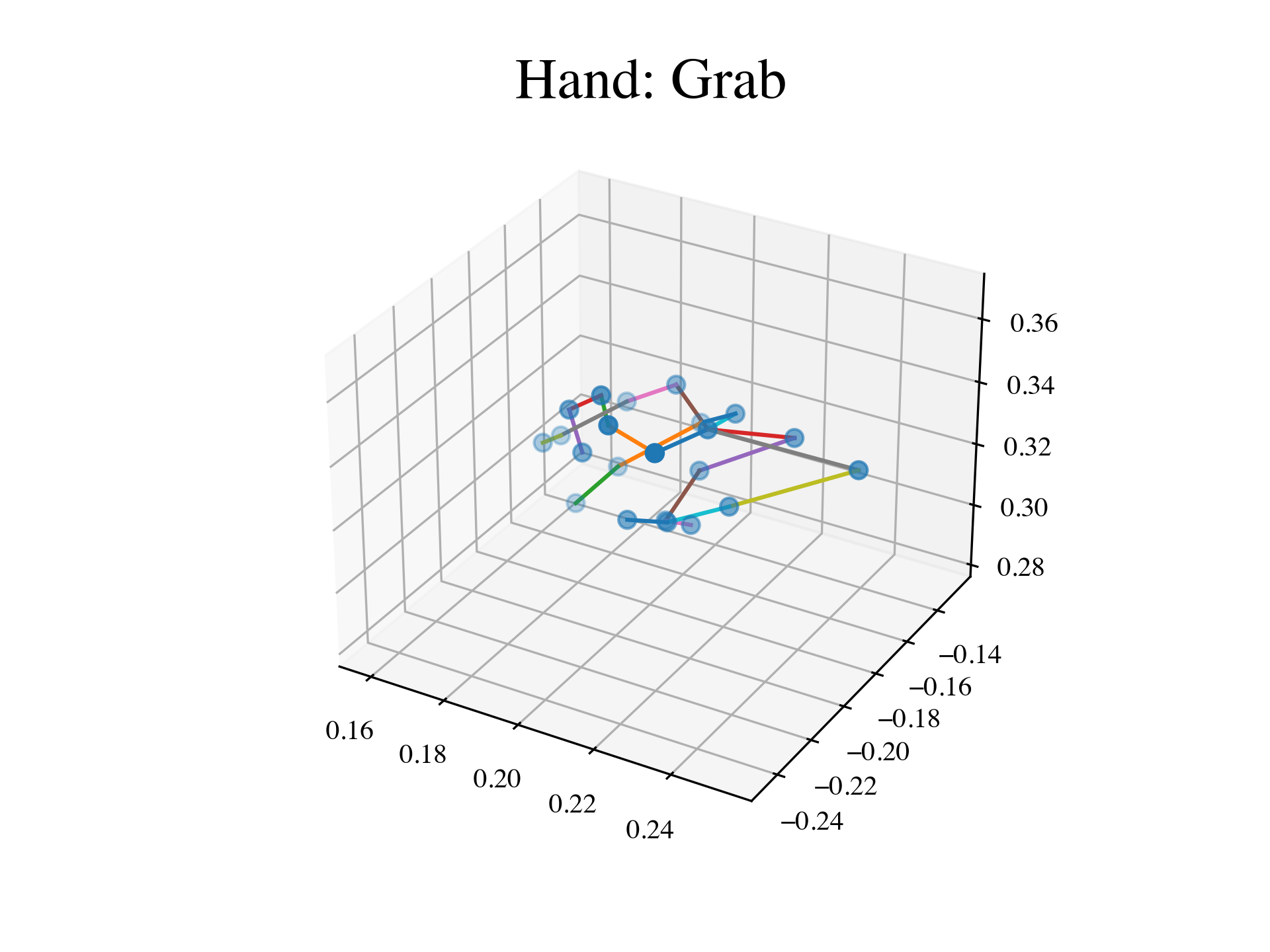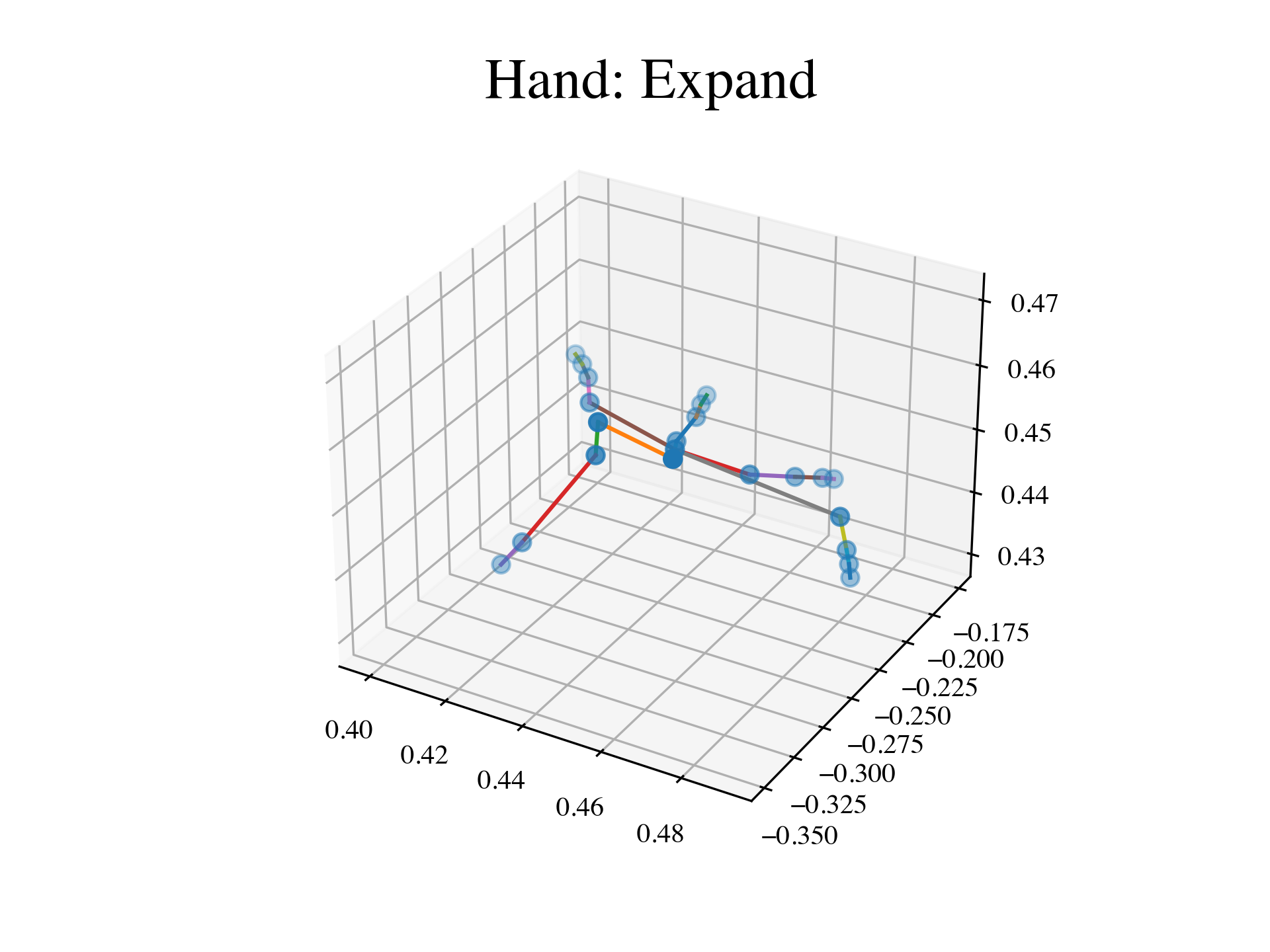# Tutorial: Classifying hands poses with Kendall shape spaces¶

In this tutorial, we show how to use geomstats to perform a shape data analysis. Specifically, we aim to study the difference between two groups of data: - hand poses that correspond to the action “Grab”, - hand poses heads that correspond to the action “Expand”.

We wish to investigate if there is a difference in these two groups.

The hand poses are represented as the coordinates of 22 joints in 3D:## Setup¶

 In :

import os
import sys
import warnings

sys.path.append(os.path.dirname(os.getcwd()))
warnings.filterwarnings('ignore')

 In :

import matplotlib.pyplot as plt
from mpl_toolkits.mplot3d import Axes3D

import geomstats.visualization as visualization
import geomstats.backend as gs
import geomstats.datasets.utils as data_utils
from geomstats.geometry.pre_shape import PreShapeSpace, KendallShapeMetric

visualization.tutorial_matplotlib()

INFO: Using numpy backend


### Hands shapes¶

Load the dataset of hand poses, where a hand is represented as a set of 22 landmarks - the hands joints - in 3D.

The hand poses represent two different hand poses: - Label 0: hand is in the position “Grab” - Label 1: hand is in the position “Expand”

This is a subset of the SHREC 2017 dataset [SWVGLF2017].

We load the dataset of landmarks’ sets and corresponding labels.

 In :

hands, labels, bone_list = data_utils.load_hands()

 In :

print(hands.shape)
print(labels)

(52, 22, 3)
[0 0 0 0 0 0 0 0 0 0 0 0 0 0 0 0 0 0 0 0 0 0 0 0 0 1 1 1 1 1 1 1 1 1 1 1 1
1 1 1 1 1 1 1 1 1 1 1 1 1 1 1]


We extract two hands, one corresponding to the “Grab” pose, and the other to the “Expand” pose.

 In :

label_to_str = {0: 'Grab', 1: 'Expand'}
label_to_color = {0: (102/255, 178/255, 255/255, 1.), 1: (255/255, 178/255, 102/255, 1.)}
first_grab_hand = hands[labels==0]
first_expand_hand = hands[labels==1]


We implement a function to plot one hand in 3D.

 In :

def plot_hand(hand, bone_list):
fig = plt.figure()
ax = plt.axes(projection="3d")

x = hand[:, 0]
y = hand[:, 1]
z = hand[:, 2]

sc = ax.scatter(x, y, z, s=40)
for bone in bone_list:
start_bone_idx = bone
end_bone_idx = bone
ax.plot(
xs=[x[start_bone_idx], x[end_bone_idx]],
ys=[y[start_bone_idx], y[end_bone_idx]],
zs=[z[start_bone_idx], z[end_bone_idx]],
)


We plot two examples of hands.

 In :

%matplotlib notebook

plot_hand(first_grab_hand, bone_list)
plt.title(f"Hand: {label_to_str}");In :

plot_hand(first_expand_hand, bone_list)
plt.title(f"Hand: {label_to_str}");We want to investigate if there is a difference between these two groups of shapes - grab versus expand - or if the main difference is merely relative to the global size of the landmarks’ sets.

 In :

m_ambient = 3
k_landmarks = 22

preshape = PreShapeSpace(m_ambient=m_ambient, k_landmarks=k_landmarks)
matrices_metric = preshape.embedding_metric

sizes = matrices_metric.norm(preshape.center(hands))

plt.figure(figsize=(6, 4))
for label, col in label_to_color.items():
label_sizes = sizes[labels==label]
plt.hist(label_sizes, color=col, label=label_to_str[label], alpha=0.5, bins=10)
plt.axvline(gs.mean(label_sizes),  color=col)
plt.legend(fontsize=14)
plt.title('Hands sizes', fontsize=14);